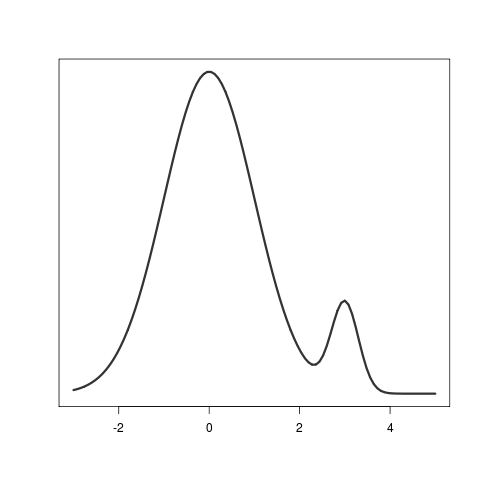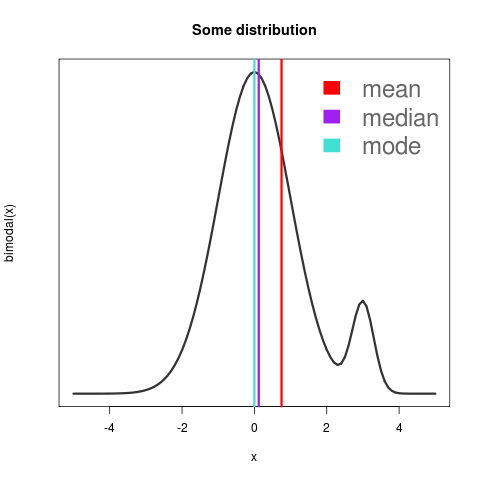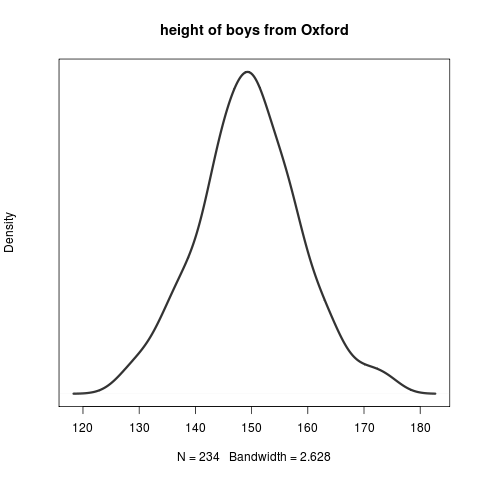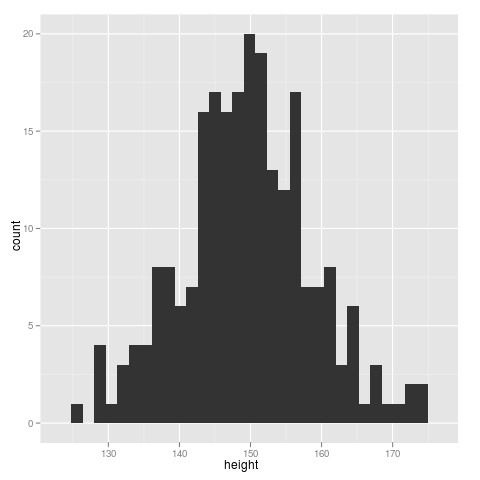Want to share your content on R-bloggers? click here if you have a blog, or here if you don't.

If I google for “probability distribution” I find the following extremely bad picture:It’s bad because it conflates ideas and oversimplifies how variable probability distributions can generally be.

• Most distributions are not unimodal.
• Most distributions are not symmetric.
• Most distributions do not have `mean` = `median` = `mode`.
• Most distributions are not Gaussian, Poisson, binomial, or anything famous at all.• If this is the example you give to your students of “a distribution”, why in the world would they be surprised at the Central Limit Theorem? The reason it’s interesting is that things that don’t look like the above, sum to look like the above.
• People already mistakenly assume that everything is bell curved. Don’t reinforce the notion!
` `

Here is a better picture to use in exposition. In `R` I defined

`bimodal `.

That’s what you see here, plotted with `plot( bimodal, -3, 5, lwd=3, col="#333333", yaxt="n" )`.Here’s how I calculated the mean, median, and mode:

• mean is the most familiar  . To calculate this in `R` I defined `bimodal.x ` and did `integrate(bimodal.x, lower=-Inf, upper=Inf)`.

The output is `.75`, that’s the mean.

• mode is the x where the highest point is. That’s obviously zero. In fancy scary notation one writes “the argument of the highest probability”
• median is the most useful but also the hardest one to write the formulaic definition. Median has 50% of the probability mass to the left and 50% of the probability mass to the right. So In `R` I had to plug in lots of values to `integrate( bimodal, lower = -Inf, upper = ... )` and `integrate( bimodal, upper = Inf, lower = ...)` until I got them to be equal. I could have been a little smarter and tried to make the difference equal zero but the way I did it made sense and was quick enough.

The answer is roughly `.12`.

```> integrate( bimodal, lower = -Inf, upper = .12 )
1.643275 with absolute error < 1.8e-08
> integrate( bimodal, upper = Inf, lower = .12 )
1.606725 with absolute error < 0.0000027```

(I could have even found the exact value in Excel using the solver. But I felt lazy, please excuse me.)Notice that I drew the numbers as vertical lines rather than points on the curve. And I eliminated the vertical axis labels. That’s because the mean, median, and mode are all x values and have nothing whatever to do with the vertical value. If I could have figured out how to draw a coloured dot at the bottom, I would have. You could also argue that I should have shown more humps or made the mean and median diverge even more.

Here’s how I drew the above:

```png("some bimodal dist.png")
leg.text ```

Lastly, it’s not that hard in the computer era to get an actual distribution drawn from facts. The `nlme` package has actually recorded heights of boys from Oxford:

```require(nlme); data(Oxboys); plot( density( Oxboys\$height), main = "height of boys from Oxford", yaxt="n", lwd=3, col="#333333")
```

and boom:or in histogram form with `ggplot`, run `require(ggplot2); qplot( data = Oxboys, x = height )` and get:the heights look Gaussian-ish, without mistakenly giving students the impression that real-world data follows perfect bell-shaped patterns.